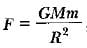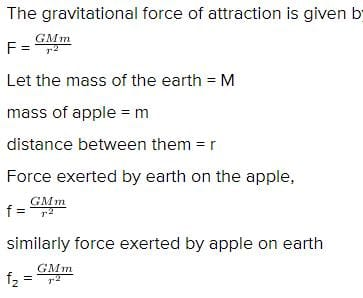Important Questions (1 mark): Gravitation

# Important Questions (1 mark): Gravitation - Class 9

Test Description

## 20 Questions MCQ Test Online MCQ Tests for Class 9 - Important Questions (1 mark): Gravitation

Important Questions (1 mark): Gravitation for Class 9 2023 is part of Online MCQ Tests for Class 9 preparation. The Important Questions (1 mark): Gravitation questions and answers have been prepared according to the Class 9 exam syllabus.The Important Questions (1 mark): Gravitation MCQs are made for Class 9 2023 Exam. Find important definitions, questions, notes, meanings, examples, exercises, MCQs and online tests for Important Questions (1 mark): Gravitation below.
Solutions of Important Questions (1 mark): Gravitation questions in English are available as part of our Online MCQ Tests for Class 9 for Class 9 & Important Questions (1 mark): Gravitation solutions in Hindi for Online MCQ Tests for Class 9 course. Download more important topics, notes, lectures and mock test series for Class 9 Exam by signing up for free. Attempt Important Questions (1 mark): Gravitation | 20 questions in 10 minutes | Mock test for Class 9 preparation | Free important questions MCQ to study Online MCQ Tests for Class 9 for Class 9 Exam | Download free PDF with solutions
 1 Crore+ students have signed up on EduRev. Have you?
Important Questions (1 mark): Gravitation - Question 1

### The universal law of gravitation was postulated by

Important Questions (1 mark): Gravitation - Question 2

### There is no atmosphere on moon as

Important Questions (1 mark): Gravitation - Question 3

### The constant G

Detailed Solution for Important Questions (1 mark): Gravitation - Question 3

The small size of the gravitational constant G  tells you that  the gravitational force is actually quite weak compared to other known  forces  like the electric, magnetic and  nuclear forces.

Important Questions (1 mark): Gravitation - Question 4

Acceleration due to gravity is

Important Questions (1 mark): Gravitation - Question 5

If the radius of earth were to shrink by 1%, its mass remaining the same, the acceleration due to gravity on earth’s surface would

Important Questions (1 mark): Gravitation - Question 6

An apple and a stone dropped from certain height accelerates

Important Questions (1 mark): Gravitation - Question 7

The gravitational force between two bodies does not depend on

Important Questions (1 mark): Gravitation - Question 8

Weight of 1 kg is

Important Questions (1 mark): Gravitation - Question 9

10 kg wt equals

Important Questions (1 mark): Gravitation - Question 10

A body at rest having uniform acceleration is

Detailed Solution for Important Questions (1 mark): Gravitation - Question 10

The correct option is Option C.

A body at rest having uniform acceleration is any body thrown vertically up against gravity.

When a body is thrown vertically upwards, at the highest point only velocity is zero because the acceleration due to gravitational force is acting downward continuously and that is the reason for velocity being zero at the highest point, hence velocity is zero because of the acceleration.

Important Questions (1 mark): Gravitation - Question 11

At which of the following locations is the value of g largest?

Important Questions (1 mark): Gravitation - Question 12

acceleration due to gravity varies with

Important Questions (1 mark): Gravitation - Question 13

Two objects of different masses falling freely near the surface of moon would

Detailed Solution for Important Questions (1 mark): Gravitation - Question 13

When an object is under free fall, its acceleration depends only on the acceleration due to gravity and mass has no role to play. Due to this; irrespective of their masses, the object under free fall with have the same velocity at any instant.

Important Questions (1 mark): Gravitation - Question 14

Two particles are placed at some distance. If the mass of each of the two particles is doubled, keeping the distance between them unchanged, the value of gravitational force between them will be

Important Questions (1 mark): Gravitation - Question 15

In the relation, the quantity  G

Important Questions (1 mark): Gravitation - Question 16

The force of attraction between two unit point masses separated by a unit distance is called

Important Questions (1 mark): Gravitation - Question 17

Two particles are placed at some distance. If mass of each of the two particles is doubled, keeping the distance between them unchanged, the value of gravitational force between them will become/remain

Important Questions (1 mark): Gravitation - Question 18

An apple falls from a tree because of gravitational attraction between the earth and apple. If F1 is the magnitude of force exerted by the earth on the apple and F2 is the magnitude of force exerted by apple on earth, then

Detailed Solution for Important Questions (1 mark): Gravitation - Question 18so we can see that f is equal to f₂

Important Questions (1 mark): Gravitation - Question 19

The weight of an object at the centre of the earth of radius R is

Detailed Solution for Important Questions (1 mark): Gravitation - Question 19

The weight of the body at the surface of the earth is equal to zero. The accelertion due to gravity is maximum on the surface of the earth and as we towards the centre of the equator it becomes zero.

The acceleration due to gravity varies due to the shape of the earth. The radius of the earth is more at the equator than at the poles.

Thus, the value of g at the centre of the earth is zero.

Important Questions (1 mark): Gravitation - Question 20

Freely falling objects weight ._______.

Detailed Solution for Important Questions (1 mark): Gravitation - Question 20

An object kept in a lift which falls freely  , weighs zero on the weighing machine , but its actual  weight is still mg. This happens  because the normal reaction force Exerted on the object in the lift is equal to mg,which in turn equals the  weight of the  object.

## Online MCQ Tests for Class 9

399 tests
Information about Important Questions (1 mark): Gravitation Page
In this test you can find the Exam questions for Important Questions (1 mark): Gravitation solved & explained in the simplest way possible. Besides giving Questions and answers for Important Questions (1 mark): Gravitation , EduRev gives you an ample number of Online tests for practice

399 tests